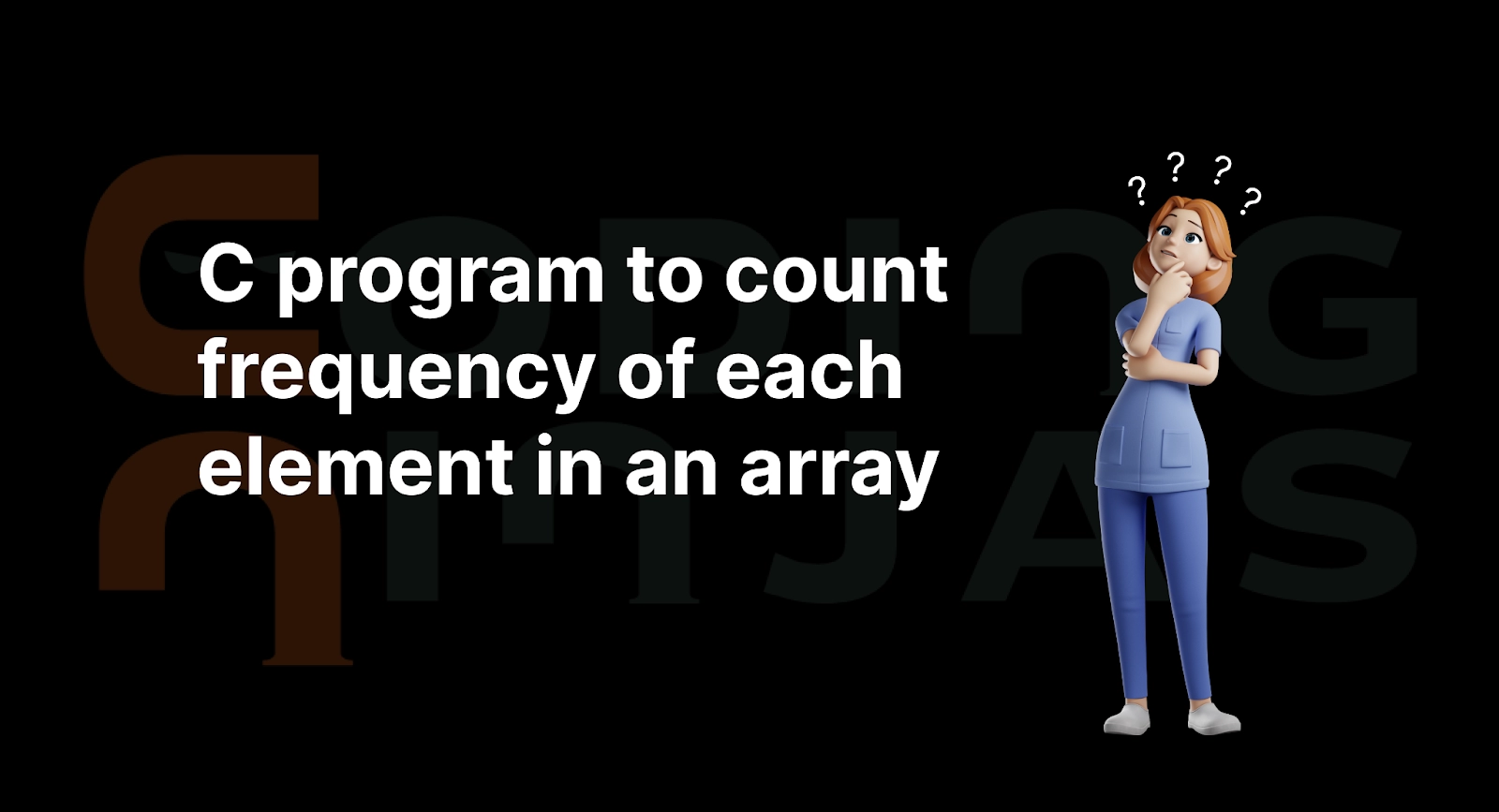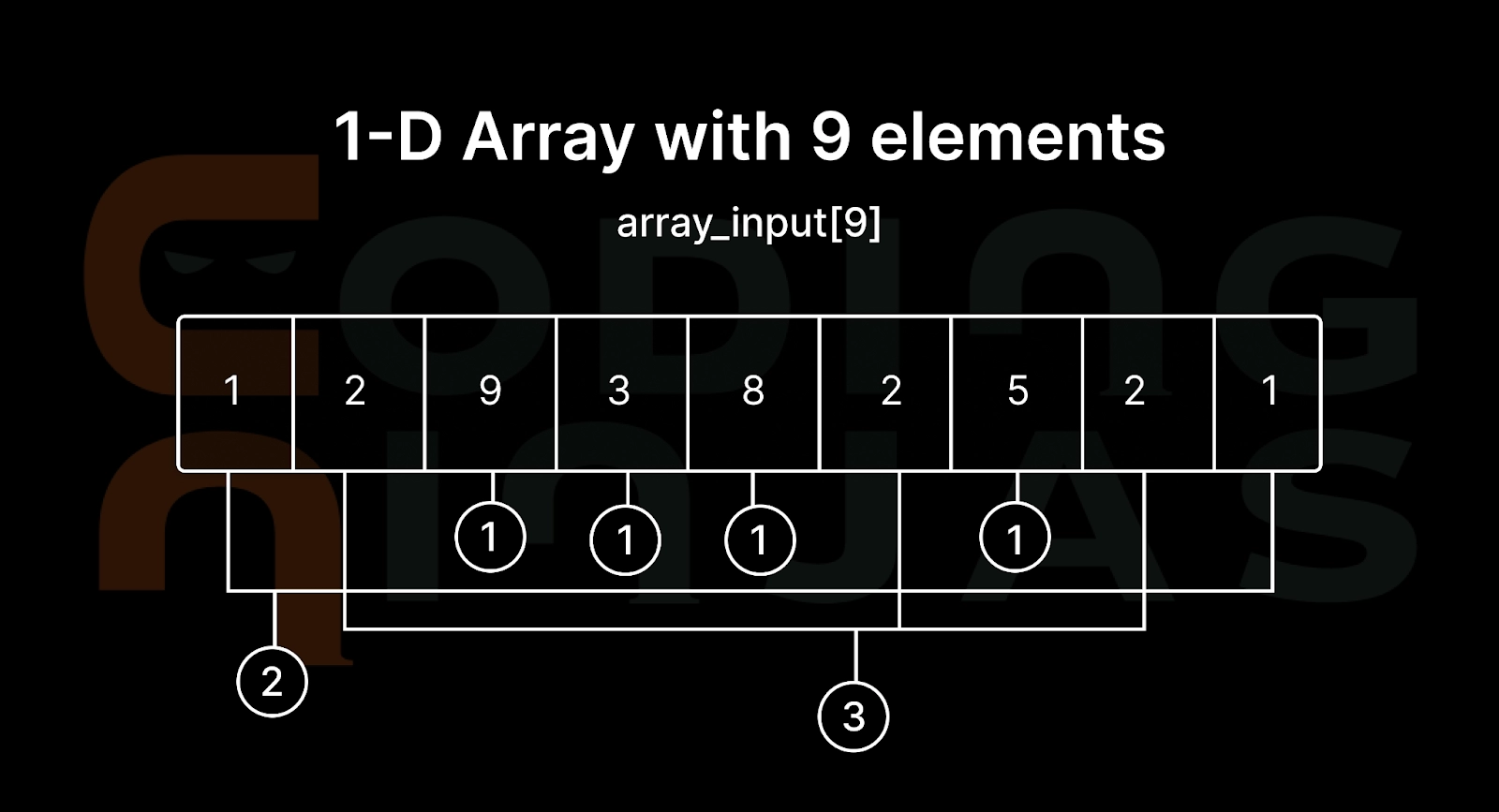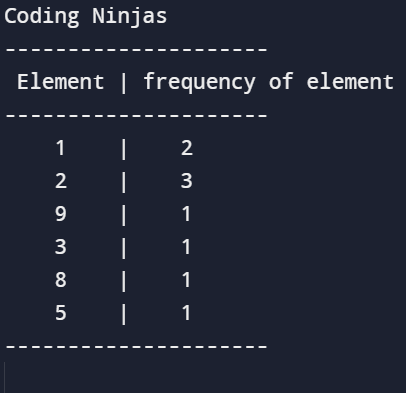New update is available. Click here to update.

# C Program to Count Frequency of Each Element in an Array

## Introduction

C language is a general-purpose computer programming language. C language was created in the 1970s by Dennis Ritchie and remains influential and very widely used.In this blog, we will cover a problem, which is a C program to count frequency of each element in an array with an example and proper explanation.

Recommended Topic, Binary to Hex Converter.

## Problem Statement

Write a C program to take input elements in the array from the user and find the frequency of each array's element.

Or

C program to count frequency of each element in an array

### Pictorial RepresentationThis image shows the count of each element present in the array. In other words, it shows the frequency of each element in the array of size 9.

We will use nested for loops to calculate the frequency of each array element. We are first calculating the frequency of the first element (array_input) and triggering the inner loop, which checks for other elements after the first element whether they are equal or not. If the elements are equal, we increase the count of the variable defined to calculate frequency and mark the index as visited.

Similarly, for every element, we are doing the same.

### Input

Input array elements: 1, 2, 9, 3, 8, 2, 5, 2, 1

### Output

The ‘C program to count frequency of each element in an array problem output will display the frequency of each element of the array.

For the above example of input array elements, output is-### Required Knowledge

Basic ‘Input-Output’, ‘If-else’, ‘For’ loop, ‘Array’ knowledge is required.

## Optimised Approach

The approach of solving the problem C program to count frequency of each element in an array is finding the frequency of each element of an array is based on the logic of finding duplicate elements in an Array.

One of the approaches is to maintain an array to store the counts of each element of the array. After that, loop through the given array and count the occurrence of array's each element as frequency and store it in array frequency.

### Algorithm

``````STEP 1: START

STEP 2: INITIALIZE array_input[] = {1, 2, 9, 3, 8, 2, 5, 2, 1}.

STEP 3: length = sizeof(array_input)/sizeof(array_input)

STEP 4: DEFINE frequency [length].

STEP 5: SET visited = -1

STEP 6: SET i = 0. REPEAT STEP 7 to 12 UNTIL i<length.

STEP 7: SET count_var = 1

STEP 8: SET j = 0. REPEAT STEP 9 and STEP 10 UNTIL j<length.

STEP 9: if(array_input[i] == array_input[j]) then
count_var++
frequency [j] = visited

STEP 10: j= j+1

STEP 11: if(frequency [i] != visited) then
frequency [i] = count_var

STEP 12: i=i+1

STEP 13: PRINT "------------"

STEP 14: PRINT "Element | frequency of element"

STEP 15: PRINT "-------------"

STEP 16: SET i = 0. REPEAT STEP 17 to 18 UNTIL i<length.

STEP 17: if(frequency [i] != visited) then
PRINT array_input[i]
PRINT | PRINT frequency [i]

STEP 18: i = i+1.

STEP 19: PRINT "-------------"

STEP 20: RETURN 0.

STEP 21: END``````

### Code

``````#include <stdio.h>

int main()
{
printf("Coding Ninjas\n");
int array_input[] = {1, 2, 9, 3, 8, 2, 5, 2, 1};

int length = sizeof(array_input)/sizeof(array_input);

int frequency [length];
int visited = -1;

for(int i = 0; i < length; i++){
int count_var = 1;
for(int j = i+1; j < length; j++){
if(array_input[i] == array_input[j]){
count_var++;
frequency [j] = visited;
}
}
if(frequency [i] != visited)
frequency [i] = count_var;
}

printf("---------------------\n");
printf(" Element | frequency of element\n");
printf("---------------------\n");
for(int i = 0; i < length; i++){
if(frequency [i] != visited){
printf("    %d", array_input[i]);
printf("    |  ");
printf("  %d\n", frequency [i]);
}
}

printf("---------------------\n");
return 0;
}   ``````

### OutputFor a good understanding, you can implement it on C online compiler for practice.

## Complexity

### Time complexity

For declaring variables and array the time complexity of O(1).

So for these line of code the time complexity is O(1)-

``````int array_input[] = {1, 2, 9, 3, 8, 2, 5, 2, 1};
int length = sizeof(array_input)/sizeof(array_input);
int frequency [length];
int visited = -1;  ``````

For nested loop the time complexity of O(n2), so for below given line of code it is O(n2)-

``````for(int i = 0; i < length; i++){
int count_var = 1;
for(int j = i+1; j < length; j++){
if(array_input[i] == array_input[j]){
count_var++;
frequency [j] = visited;
}
}
if(frequency [i] != visited)
frequency [i] = count_var;
}
``````

And single for loop have time complexity of O(n), below given code has O(n) time complexity-

``````for(int i = 0; i < length; i++){
if(frequency [i] != visited){
printf(" %d", array_input[i]);
printf(" | ");
printf(" %d\n", frequency [i]);
}
} ``````

Dropping constants (O(1)) and non-dominant terms (O(n)).

### Space complexity

The arrays (array_input and frequency) consist of 9 integer elements and <9 in the code we implemented. At max, frequency length can be equal to array_input length only if every array element appears only once. In general, an array can have any number of elements that is 'n'. So, the space occupied by the array is 4 (size of int data type) * n. Also, we have integer variables such as length, visited, and count_var. The total space occupied by the program is 4n + 12 bytes assuming 4 bytes for each variable. Since the highest order of n in the equation 4n + 12 is n, the space complexity is O(n) or linear.

As the above code has a time complexity of O(n2) and space complexity of O(n), so we will go for another method, that is, by making the elements of the array negative.

Check out this problem - First And Last Occurrences Of X

## Approach

At first, we will traverse through the given array_input and store their count at the index. We are using a hashmap of size n and the array_input with the size of n. Here, the array_input will be used as a hashmap. All n elements of the array_input are positive elements, and the frequency can be negative. But we will encounter a problem that is let ith element be a then the count of a will stored in array_input[a-1], as soon as the frequency gets stored the element will be lost. To deal with this problem, we will first replace the ith element with array_input[a-1] and then put -1 at array_input[a-1]. So basically, we are replacing the element by frequency and storing the element in the current index. If the element at array_input[a-1] is already negative, it is already replaced by a frequency, so decrement array_input[a-1].

### Algorithm

``````STEP 1: From start to end, traverse the array_input.

STEP 2: Now check if the element is less than or equal to zero. If it is negative or zeroes, you can skip it as it is frequency.

STEP 3: If the element (a=array_input[i]-1) is positive then you can check if array_input[a] is positive or not. If the element is positive that means it is the first occurence of a in the given array_input and replace array_input[i] with array_input[a] that is array_input[i]=array_input[a] and assign array_input[a] =-1. if array_input[a] is negative that means it not the first occurence of the element a and update array_input[a] with array_input[a]- and update array_input[i with array_input[i]=0;

STEP 4: Again, traverse through the array_input and print i+1 as value and array_input[i as frequency.``````

### Code

``````#include <stdio.h>
void findCounts(int *array_input, int n)
{
int i = 0;
while (i<n)
{
if (array_input[i] <= 0)
{
i++;
continue;
}
int index_of_element = array_input[i]-1;
if (array_input[index_of_element] > 0)
{
array_input[i] = array_input[index_of_element];
array_input[index_of_element] = -1;
}
else
{
array_input[index_of_element]--;
array_input[i] = 0;
i++;
}
}
printf("\nC program to count frequency of each element in an array\n");
for (int i=0; i<n; i++)
printf("%d -> %d\n", i+1, abs(array_input[i]));
}

int main()
{
int array_input[] = {1, 2, 9, 3, 8, 2, 5, 2, 1};
findCounts(array_input, sizeof(array_input)/ sizeof(array_input));

return 0;
}``````

## Complexity

### Time complexity

As O(n)  time complexity is taken by the array for single time traversal.

### Space complexity

We need no extra space with this code so the space complexity is O(1).

### What is C?

C language is a general-purpose computer programming language. C language was created in the 1970s by Dennis Ritchie and remains influential and very widely used.

### What is time complexity?

It is the time taken by the computer to run an algorithm. In other words, it shows how a function's runtime increases as the size of input increases.

### What is space complexity?

It is the amount of memory space needed by an algorithm to solve an instance of the problem as a function of the input. Or in other words, it is the memory space required in the worst case at any point of the algorithm.

### Why did we use the variable 'count_var' in the above problem?

We used the variable count_var to get each array element's number of times/frequency.

### How does the nested loop work?

Initially, if the condition is satisfied, the outer loop passes to the inner loop and executes for the inner loop until the inner loop condition becomes false. Then the second pass of the outer loop encounters the inner loop if the condition satisfies, and it continues until the condition becomes false. It will be repeated until the outer loop finishes.

## Conclusion

In this blog, we saw the C program to count frequency of each element in an array. We understood it with an example and proper explanation and if you would like to learn more, check out our articles on

Refer to our guided paths on Codestudio to learn more about DSA, Competitive Programming, JavaScript, System Design, etc. Enroll in our courses and refer to the mock test and problems available. Take a look at the interview experiences and interview bundle for placement preparations.

Do upvote our blog to help other ninjas grow.Happy Learning Ninja!